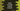# Kotlin sortedBy method example## Introduction :

Kotlin sortedBy is useful to sort collection items. You can use it to sort items in natural order or by using a selector function. It returns a list of all elements sorted. In this quick example, I will show you how to use sortedBy with examples.

### Example 1: array of integers :

Let’s consider the below example :

``````fun main() {
val givenArray = intArrayOf(10, 7, 3, 8, 1, 5, 6, 2, 9, 4)

println(givenArray.sortedBy { it })
println(givenArray.sortedBy { 10 - it })
}``````

Here, we have created one integer array givenArray with ten numbers. Next, we are sorting this array two times : the first println uses natural sorting and the second println sorts it based on the nearest value to 10. It will print the below output :

``````[1, 2, 3, 4, 5, 6, 7, 8, 9, 10]
[10, 9, 8, 7, 6, 5, 4, 3, 2, 1]``````

### Example 2: list of strings :

This example uses one list of strings :

``````fun main() {
val givenList = listOf("apple", "boy", "call", "dog", "elephant", "friday")

println(givenList.sortedBy { it })
println(givenList.sortedBy { it.length })
}``````

#### It will print :

``````[apple, boy, call, dog, elephant, friday]
[boy, dog, call, apple, friday, elephant]``````

Here, the first one sorts the list naturally and the second one sorts it based on the length.

### Example 3: list of objects :

We can use sortedBy with any custom objects as well :

``````data class Student(val marks: Int, val age: Int)

fun main() {
val studentList = listOf(Student(40, 20), Student(50, 18), Student(60, 17), Student(64, 23))

println(studentList.sortedBy { it.marks })
println(studentList.sortedBy { it.age })
}``````

Here, we have one data class students with two integer properties : marks and age. We are creating a list of four Student objects and sorting them using sortedBy.

The first one sorts it based on the value of marks and the second one sorts it based on the value of age.

#### Output :

``````[Student(marks=40, age=20), Student(marks=50, age=18), Student(marks=60, age=17), Student(marks=64, age=23)]
[Student(marks=60, age=17), Student(marks=50, age=18), Student(marks=40, age=20), Student(marks=64, age=23)]``````